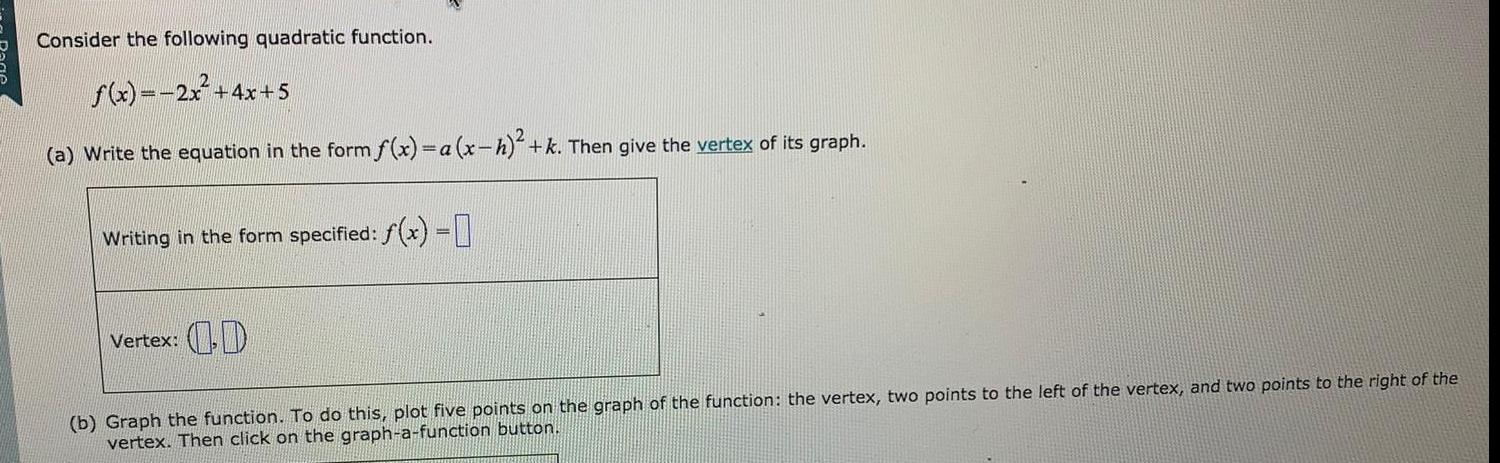Math
Parabola
Consider the following quadratic function. f(x)=2x² + 4x+5 (a) Write the equation in the form f(x)= a (x-h)² + k. Then give the vertex of its graph. (b) Graph the function. To do this, plot five points on the graph of the function: the vertex, two points to the left of the vertex, and two points to the right of the vertex. Then click on the graph-a-function button.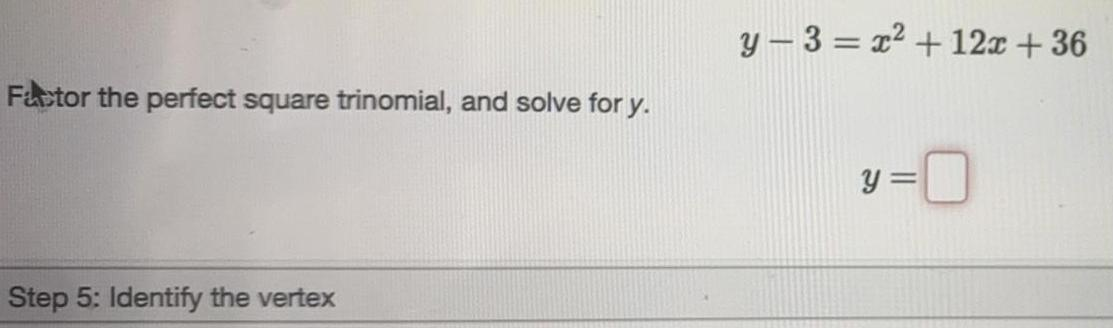Math
Parabola
y - 3 = x² + 12x +36 Factor the perfect square trinomial, and solve for y.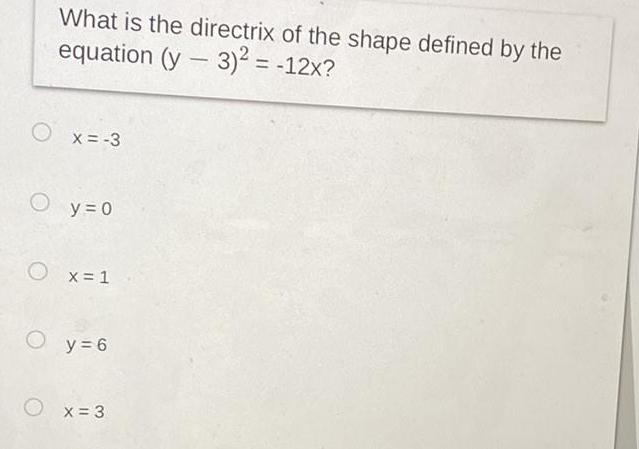Math
Parabola
O What is the directrix of the shape defined by the equation (y - 3)2 = -12x? x = -3 y = 0 Ox=1 O y = 6 x=3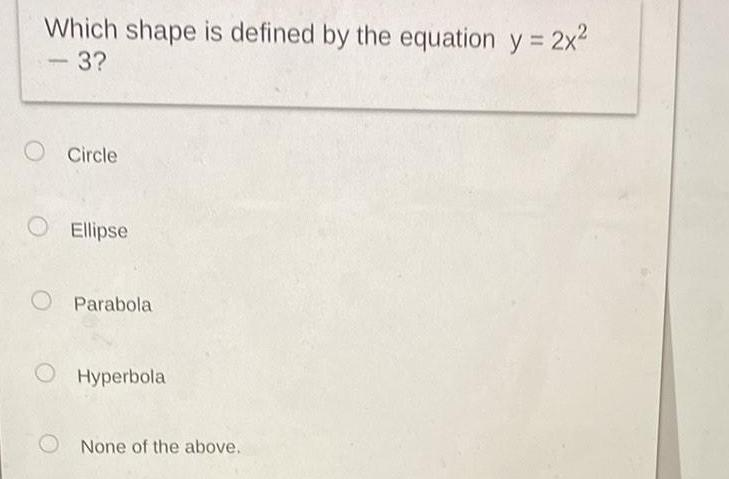Math
Parabola
Which shape is defined by the equation y = 2x² 3? - O Circle O Ellipse Parabola Hyperbola None of the above.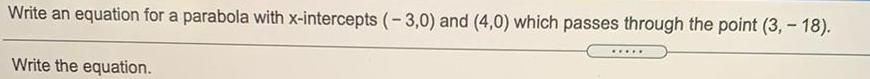Math
Parabola
Write an equation for a parabola with x-intercepts (-3,0) and (4,0) which passes through the point (3,- 18). Write the equation. *****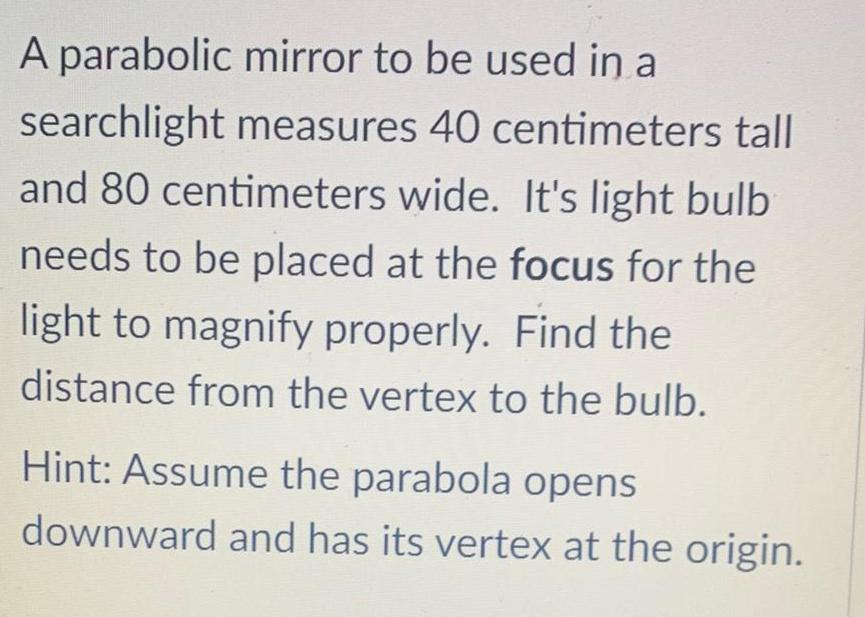Math
Parabola
A parabolic mirror to be used in a searchlight measures 40 centimeters tall and 80 centimeters wide. It's light bulb needs to be placed at the focus for the light to magnify properly. Find the distance from the vertex to the bulb. Hint: Assume the parabola opens downward and has its vertex at the origin.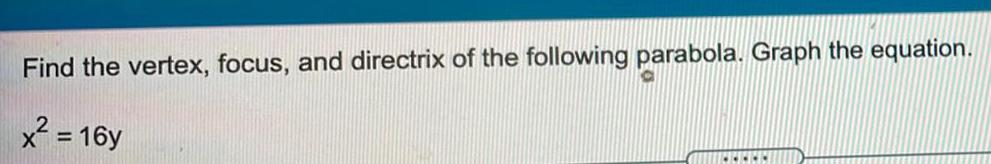Math
Parabola
Find the vertex, focus, and directrix of the following parabola. Graph the equation. x² = 16y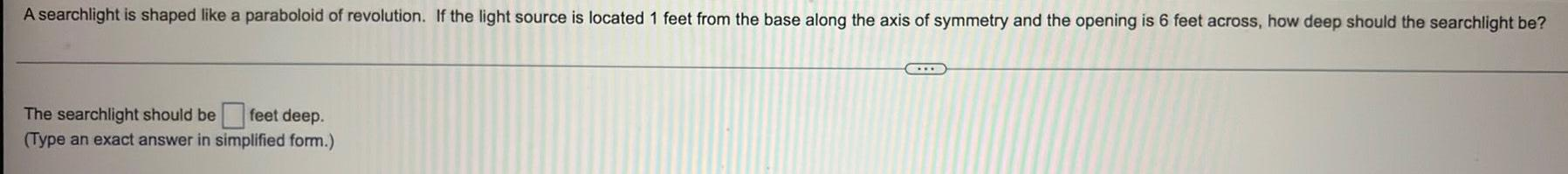Math
Parabola
A searchlight is shaped like a paraboloid of revolution. If the light source is located 1 feet from the base along the axis of symmetry and the opening is 6 feet across, how deep should the searchlight be? The searchlight should be feet deep. (Type an exact answer in simplified form.) ***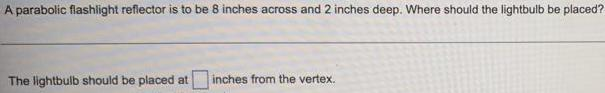Math
Parabola
A parabolic flashlight reflector is to be 8 inches across and 2 inches deep. Where should the lightbulb be placed? The lightbulb should be placed at inches from the vertex.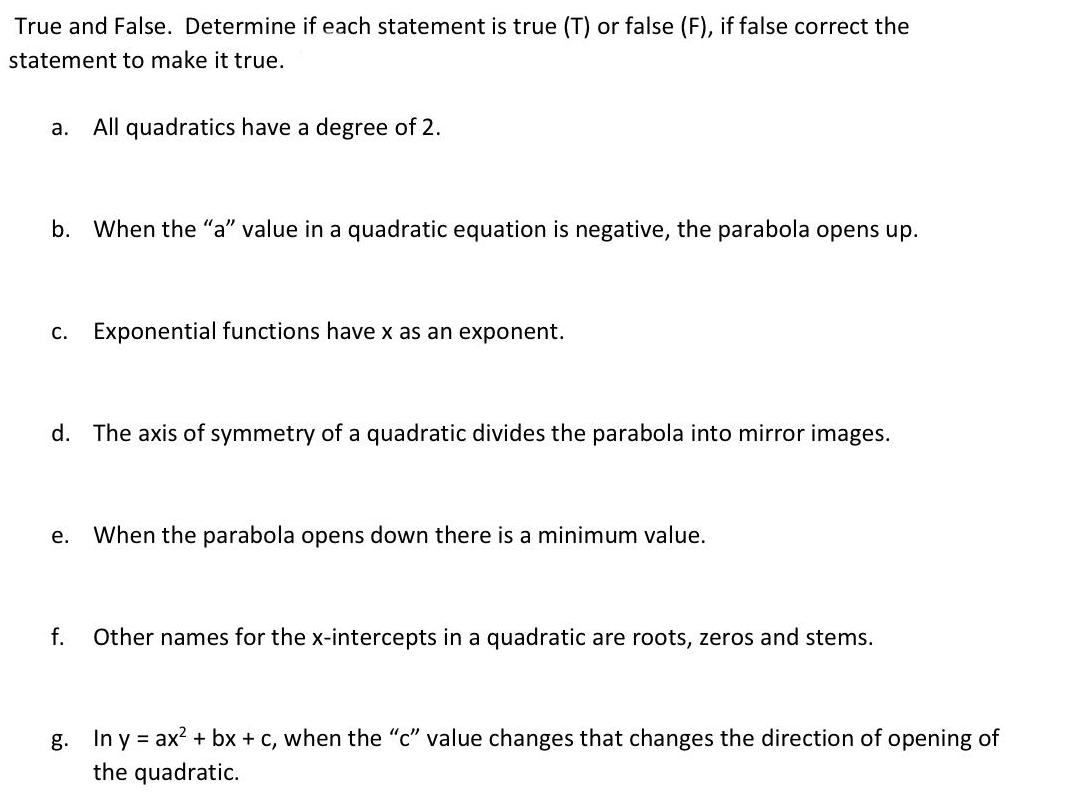Math
Parabola
True and False. Determine if each statement is true (T) or false (F), if false correct the statement to make it true. a. All quadratics have a degree of 2. b. When the "a" value in a quadratic equation is negative, the parabola opens up. c. Exponential functions have x as an exponent. d. The axis of symmetry of a quadratic divides the parabola into mirror images. e. When the parabola opens down there is a minimum value. f. Other names for the x-intercepts in a quadratic are roots, zeros and stems. g. In y = ax² + bx + c, when the "c" value changes that changes the direction of opening of the quadratic.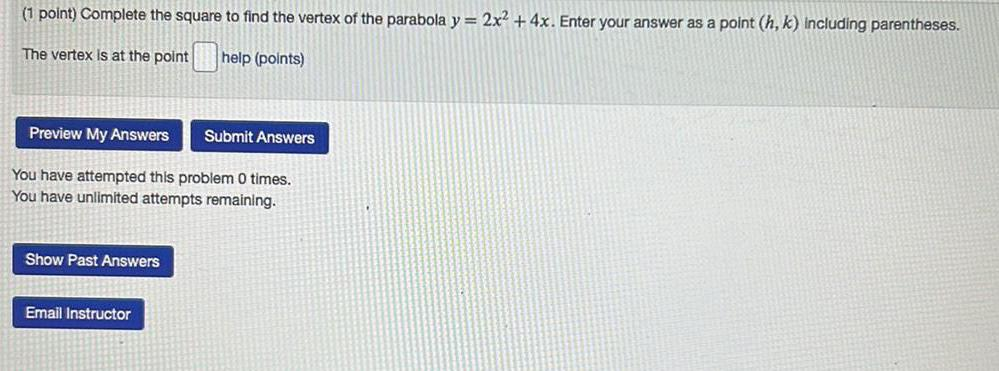Math
Parabola
Complete the square to find the vertex of the parabola y = 2x² + 4x. Enter your answer as a point (h, k) including parentheses.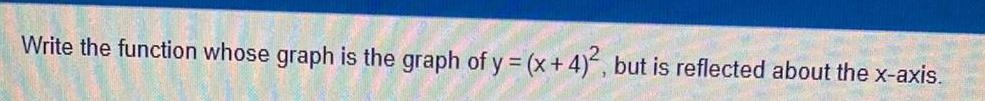Math
Parabola
Write the function whose graph is the graph of y = (x+4)², but is reflected about the x-axis.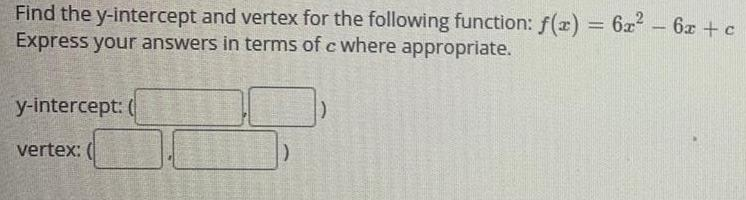Math
Parabola
Find the y-intercept and vertex for the following function: f(x) = 6x² - 6x + c Express your answers in terms of c where appropriate.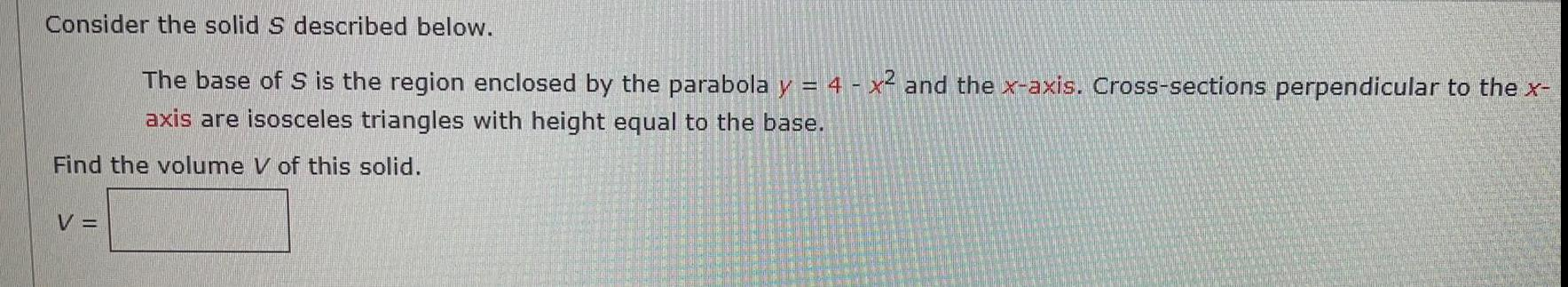Math
Parabola
Consider the solid S described below. The base of S is the region enclosed by the parabola y = 4 - x² and the x-axis. Cross-sections perpendicular to the x- axis are isosceles triangles with height equal to the base. Find the volume V of this solid. V =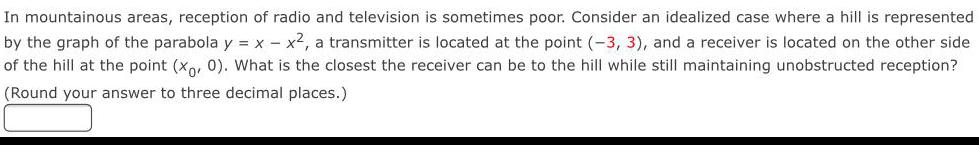Math
Parabola
In mountainous areas, reception of radio and television is sometimes poor. Consider an idealized case where a hill is represented by the graph of the parabola y = x - x², a transmitter is located at the point (-3, 3), and a receiver is located on the other side of the hill at the point (x0, 0). What is the closest the receiver can be to the hill while still maintaining unobstructed reception? (Round your answer to three decimal places.)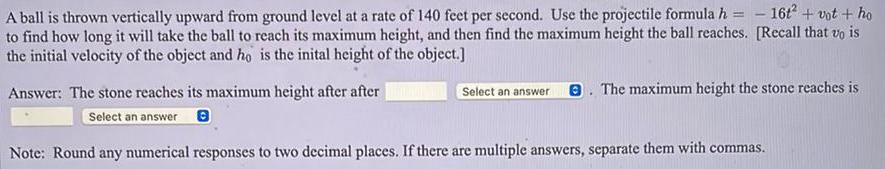Math
Parabola
A ball is thrown vertically upward from ground level at a rate of 140 feet per second. Use the projectile formula h = - 16t² + vot + ho to find how long it will take the ball to reach its maximum height, and then find the maximum height the ball reaches. [Recall that vo is the initial velocity of the object and ho is the inital height of the object.] Answer: The stone reaches its maximum height after after Select an answer The maximum height the stone reaches is Select an answer Note: Round any numerical responses to two decimal places. If there are multiple answers, separate them with commas.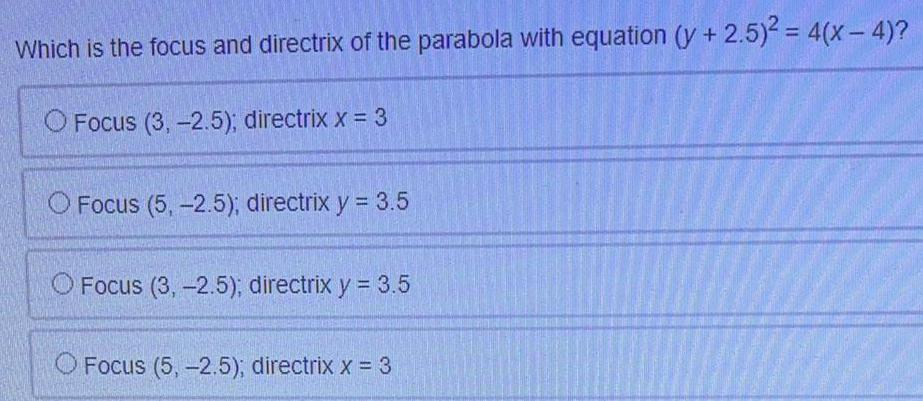Math
Parabola
Which is the focus and directrix of the parabola with equation (y + 2.5)² = 4(x-4)? Focus (3,-2.5); directrix x = 3 Focus (5,-2.5); directrix y = 3.5 Focus (3,-2.5); directrix y = 3.5 Focus (5,-2.5); directrix x = 3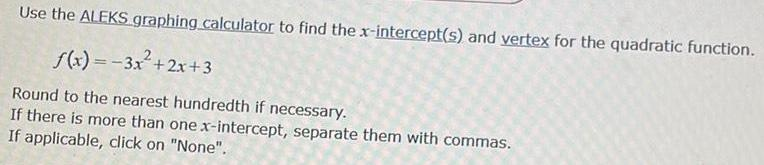Math
Parabola
Use the ALEKS graphing calculator to find the x-intercept(s) and vertex for the quadratic function. f(x) = -3x²+2x+3 Round to the nearest hundredth if necessary. If there is more than one x-intercept, separate them with commas. If applicable, click on "None".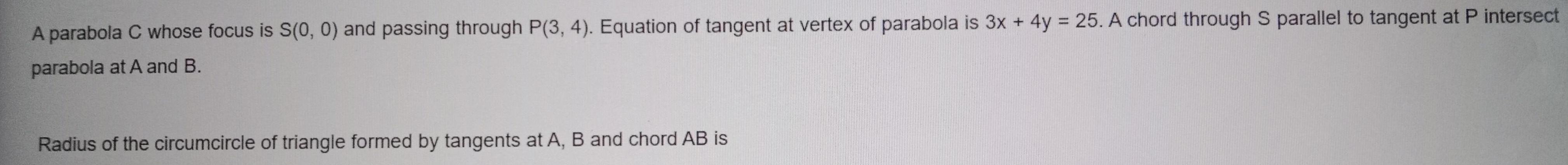Math
Parabola
A parabola C whose focus is S(0, 0) and passing through P(3, 4). Equation of tangent at vertex of parabola is 3x + 4y = 25. A chord through S parallel to tangent at P intersect parabola at A and B. Radius of the circumcircle of triangle formed by tangents at A, B and chord AB is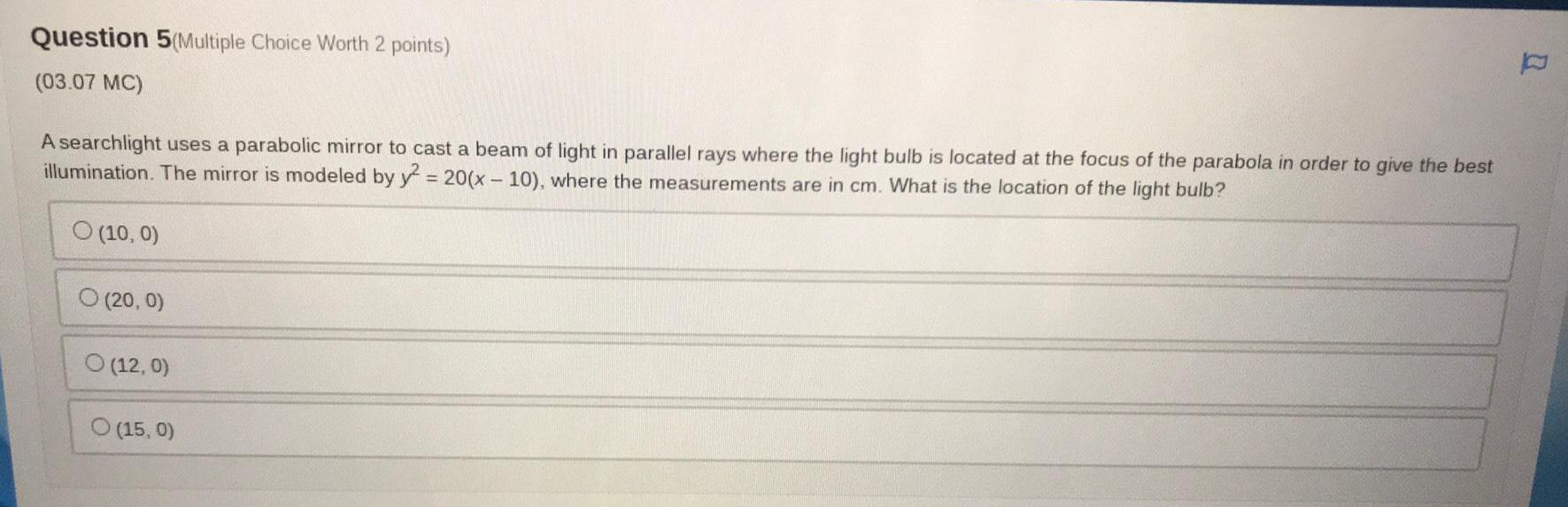Math
Parabola
A searchlight uses a parabolic mirror to cast a beam of light in parallel rays where the light bulb is located at the focus of the parabola in order to give the best illumination. The mirror is modeled by y² = 20(x - 10), where the measurements are in cm. What is the location of the light bulb? (10,0) (20, 0) (12, 0) (15, 0)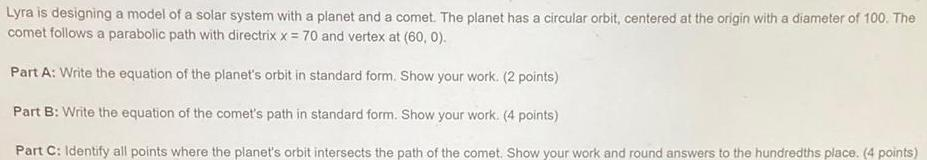Math
Parabola
Lyra is designing a model of a solar system with a planet and a comet. The planet has a circular orbit, centered at the origin with a diameter of 100. The comet follows a parabolic path with directrix x = 70 and vertex at (60, 0). Part A: Write the equation of the planet's orbit in standard form. Show your work. Part B: Write the equation of the comet's path in standard form. Show your work. Part C: Identify all points where the planet's orbit intersects the path of the comet. Show your work and round answers to the hundredths place.